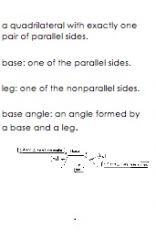• Shuffle
Toggle On
Toggle Off
• Alphabetize
Toggle On
Toggle Off
• Front First
Toggle On
Toggle Off
• Both Sides
Toggle On
Toggle Off
Toggle On
Toggle Off
Front

## Card Range To Study

throughPlay buttonPlay buttonProgress

1/29

Click to flip

Use LEFT and RIGHT arrow keys to navigate between flashcards;

Use UP and DOWN arrow keys to flip the card;

H to show hint;

 diagonal of a polygon a segment that connects any two nonconsecutive vertices. Interior Sum Theorem If a convex polygon has n sides and S is the sum of the measures of its interior angles, then S = 180 (n - 2). Exterior Sum Theorem If a polygon is convex, then the sum of the measures of the exterior angles, one at each vertex, is 360 degrees. parallelogram a quadrilateral with both pairs of opposite sides parallel. Symbols: ▱ABCD Opposite sides of a parallelogram are... ...congruent. Opposite angles of a parallelogram are... ...congruent. Consecutive angles in a parallelogram are... ...supplementary. If a parallelogram has one right angle, then... ... it has 4 right angles. Diagonals of parallelograms... ...bisects each other. Each diagonal of a parallelogram separates the parallelogram into... ...2 congruent triangles. If both pairs of opposite sides of a quadrilateral are congruent, then the quadrilateral is ... ...a parallelogram. If both pairs of opposite angles of a quadrilateral are congruent, then the quadrilateral is... ...a parallelogram. If the diagonals of a quadrilateral bisect each other, then the quadrilateral is... ...a parallelogram. If one pair of opposite sides of a quadrilateral is both parallel and congruent, then the quadrilateral... ...a parallelogram. rectangle a parallelogram with 4 right angles If a parallelogram is a rectangle, then the diagonals... ...are congruent. If the diagonals of a parallelogram are congruent, then... ...it is a rectangle. rhombus a quadrilateral with all 4 sides congruent. The diagonals of a rhombus are... ...perpendicular. If the diagonals of a parallelogram are perpendicular, then the parallelogram is... ...a rhombus. Each diagonal of a rhombus... ...bisects a pair of opposite angles. square a quadrilateral that is both a rhombus and a rectangle. kite a quadrilateral with exactly one pair of parallel sides. trapezoidisosceles trapezoid a trapezoid with congruent legs Both pairs of base angles of an isosceles trapezoid are... ...congruent. The diagonals of an isosceles trapezoid are... ...congruent. median or midsegment of a trapezoid the segment that joins the midpoint of the legs of a trapezoid. The median of a trapezoid is ___?____, and its measure is ____?____. The median is parallel to the bases, and its measure is one-half of the sum of the measures of the bases.